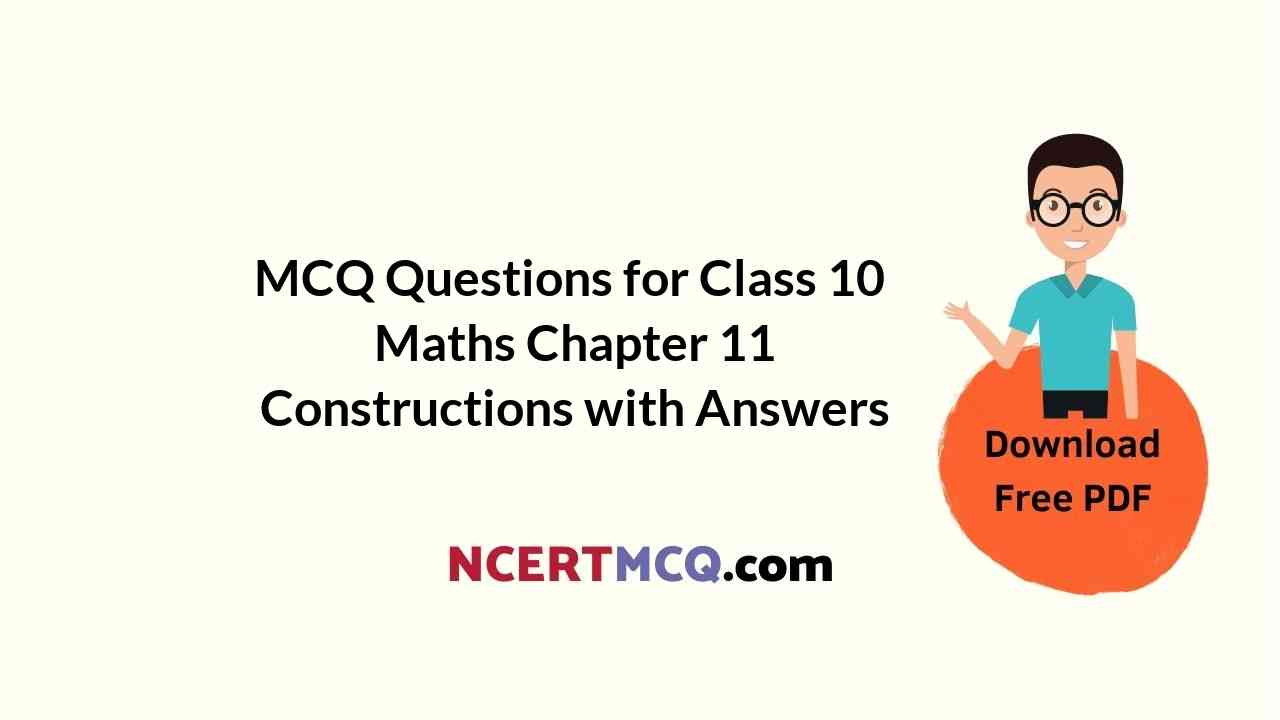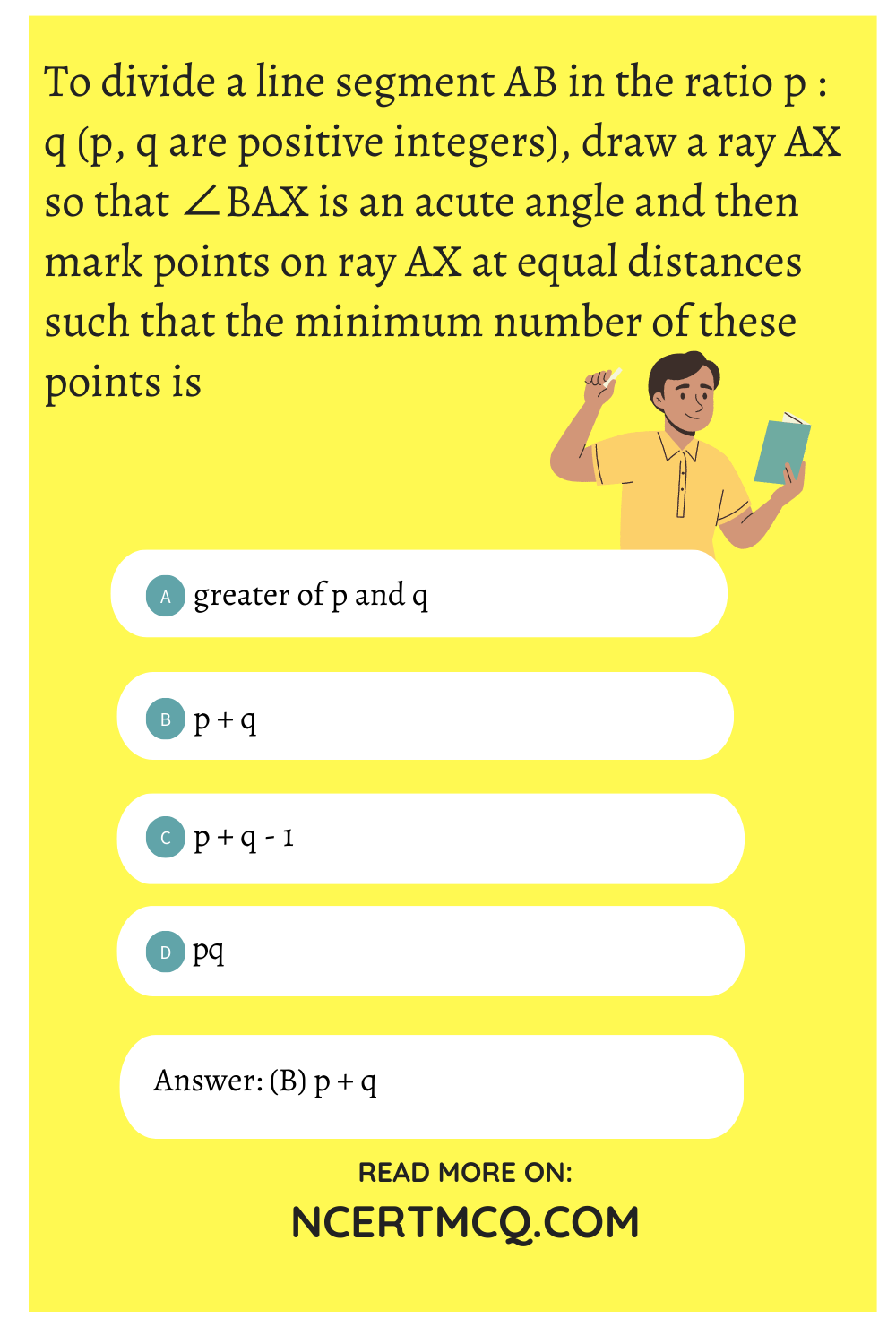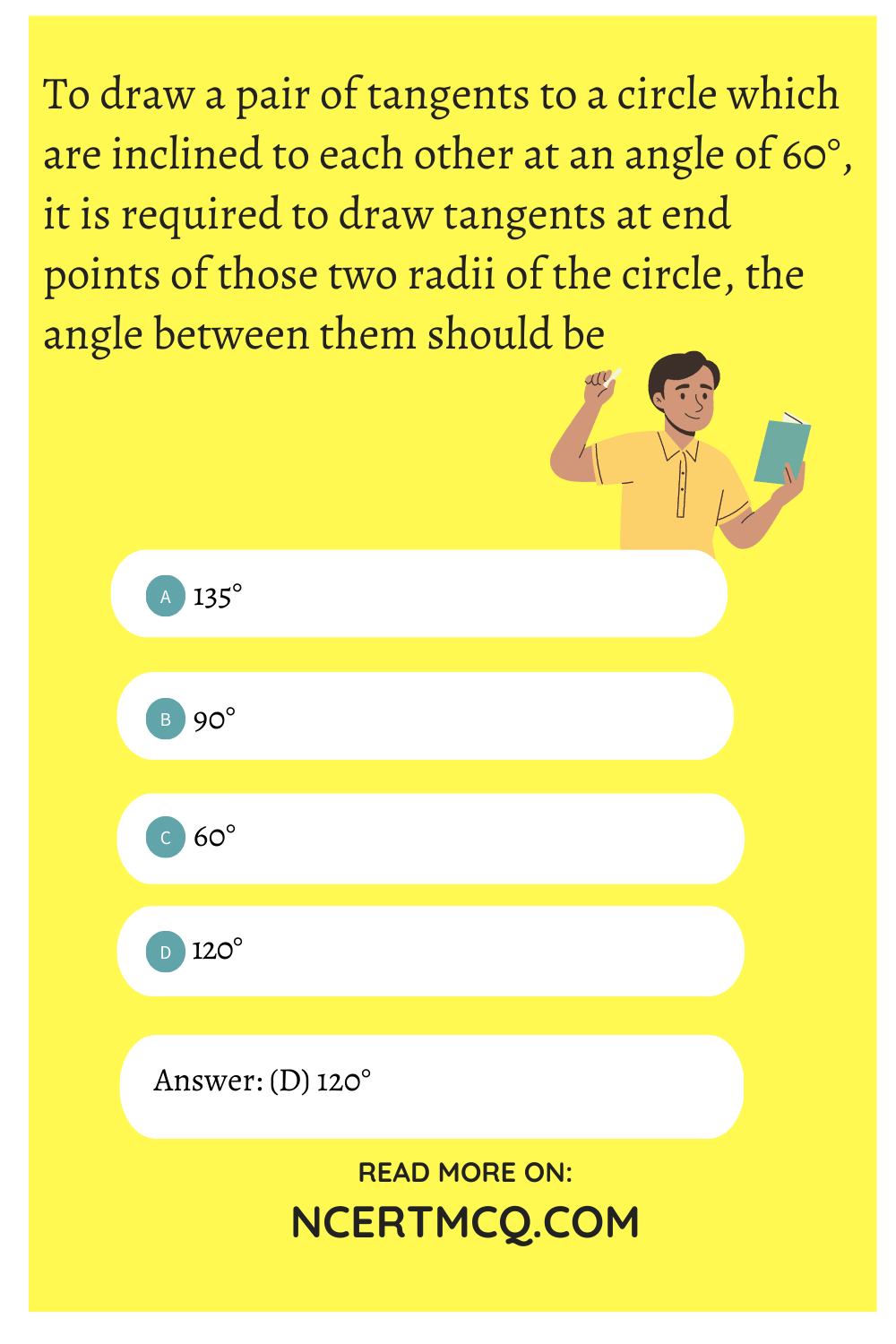Check the below NCERT MCQ Questions for Class 10 Maths Chapter 11 Constructions with Answers Pdf free download. MCQ Questions for Class 10 Maths with Answers were prepared based on the latest exam pattern. We have provided Constructions Class 10 Maths MCQs Questions with Answers to help students understand the concept very well. https://ncertmcq.com/mcq-questions-for-class-10-maths-with-answers/

Students can also refer to NCERT Solutions for Class 10 Maths Chapter 11 Constructions for better exam preparation and score more marks.

## Constructions Class 10 MCQs Questions with Answers

MCQ Questions For Class 10 Maths Chapter 11 Question 1.
To divide a line segment AB in the ratio p : q (p, q are positive integers), draw a ray AX so that ∠BAX is an acute angle and then mark points on ray AX at equal distances such that the minimum number of these points is
(a) greater of p and q
(b) p + q
(c) p + q – 1
(d) pqConstruction Class 10 MCQ Question 2.
To draw a pair of tangents to a circle which are inclined to each other at an angle of 35°. It is required to draw tangents at the end points of those two radii of the circle, the angle between which is
(a) 105°
(b) 70°
(c) 140°
(d) 145°

MCQ On Constructions Class 10 Question 3.
To divide a line segment AB in the ratio 5 : 7, first a ray AX is drawn so that ∠BAX is an acute angle and then at equal distances points are marked on the ray AX such that the minimum number of these points is
(a) 8
(b) 10
(c) 11
(d) 12

Class 10 Maths Chapter 11 MCQ Question 4.
To divide a line segment AB in the ratio 4 : 7, ray AX is drawn first such that ∠BAX is an acute angle and then points A1, A2, A3,……… are located at equal distances on the ray AX and the point B is joined to
(a) A12
(b) A11
(c) A10
(d) A9

Construction MCQ Class 10 Question 5.
To divide a line segment AB in the ratio 5 : 6, draw a ray AX such that ∠BAX is an acute angle, then draw a ray B4 parallel to AX and the points A1, A2, A3, …….. and B1, B2, B3,………. are located at equal distances on ray AX and B4, respectively. Then the points joined are
(a) A5 and B6
(b) A6 and B5
(c) A4 and B5
(d) A5 and B4

Constructions Class 10 MCQ With Answers Question 6.
To construct a triangle similar to a given ΔABC with its sides $$\frac{3}{7}$$ of the corresponding sides of ΔABC, first draw a ray BX such that ∠CBX is an acute angle and X lies on the opposite side of A with respect to BC. Then locate points B1, B2, B3, on BX at equal distances and next step is to join
(a) B10 to C
(b) B3 to C
(c) B7 to C
(d) B4 to C

MCQ Of Chapter 11 Maths Class 10 Question 7.
To construct a triangle similar to a given ΔABC with its sides $$\frac{8}{5}$$ of the corresponding sides of ΔABC draw a ray BX such that ∠CBX is an acute angle and X is on the opposite side of A with respect to BC. Then minimum number of points to be located at equal distances on ray BX is
(a) 5
(b) 8
(c) 13
(d) 3

Question 8.
To draw a pair of tangents to a circle which are inclined to each other at an angle of 60°, it is required to draw tangents at end points of those two radii of the circle, the angle between them should be
(a) 135°
(b) 90°
(c) 60°
(d) 120°